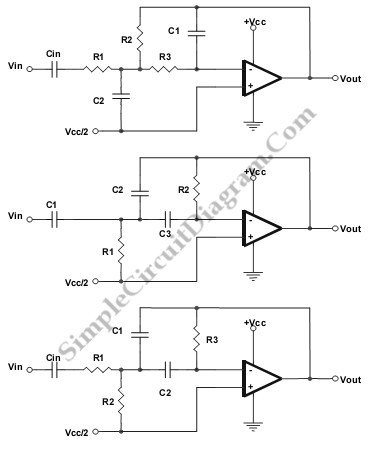# Low-, Band-, and High-Pass Single Supply Multiple Feedback Filter

This is Low-, Band-, and High-Pass Single Supply Multiple Feedback Filter circuit. This circuit uses MFB topology, which has some advantages and disadvantages. The advantages of MFB topology are low cost, easy to implement and very versatile. The disadvantage of MFB topology is it has complex calculation. This circuit uses a unity gain Butterworth, so the circuit will give a close approximation.  Here is the circuit:LOW PASS:

unity gain Butterworth

R1 = R2 = R/squareroot(2)

R3 = R/(2*squareroot(2) )

C1 = C

C2 = 4C

Fo = 1/(2pi RC)

HIGH PASS

Unity Gain Butterworth

Fo = 1/(2 piRC)

C1 = C2 = C3 = C

R1 = 0.47R

R2 = 2.1R

BAND PASS

Gain = 2.3 dB

R3 = 100R

R2 = 0.001R

R1 = 10R

C2 = C

C1 = 10C

Fo = 1/(2.32 piRC)

[Source: Texas Instruments Application Note]